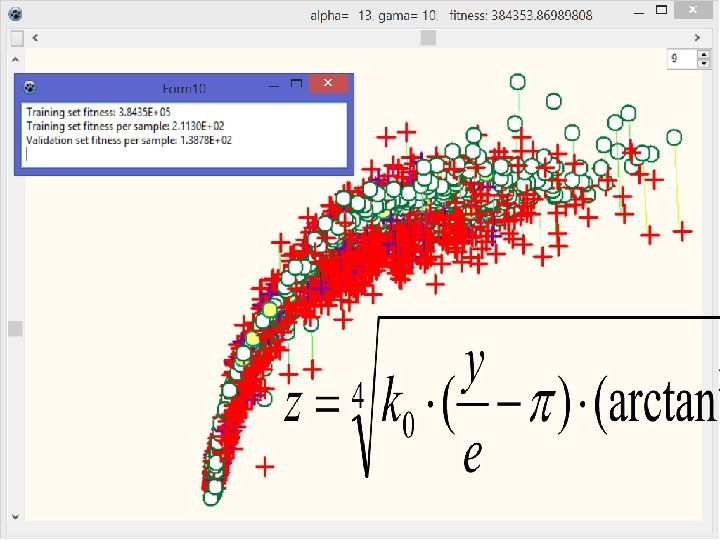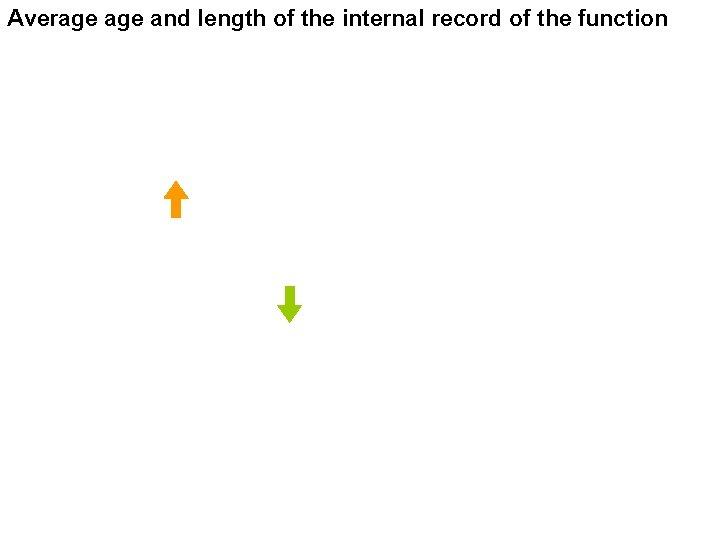# Genetic algorithms A genetic algorithm is a heuristic

• Slides: 72Genetic algorithmsA genetic algorithm is a heuristic search method used for finding optimized solutions to search problems based on theory of natural selection and the evolutionary biology. More info: Mitchell, Melanie (1996): An Introduction to Genetic Algorithms. Cambridge, MA: MIT Press. ISBN 9780585030944Source: Wikipedia. org Till now, only translation form DNA to RNA and from codons (triplets) to protein bases are fully understand (64 possible codes, but only 20 proteins coded)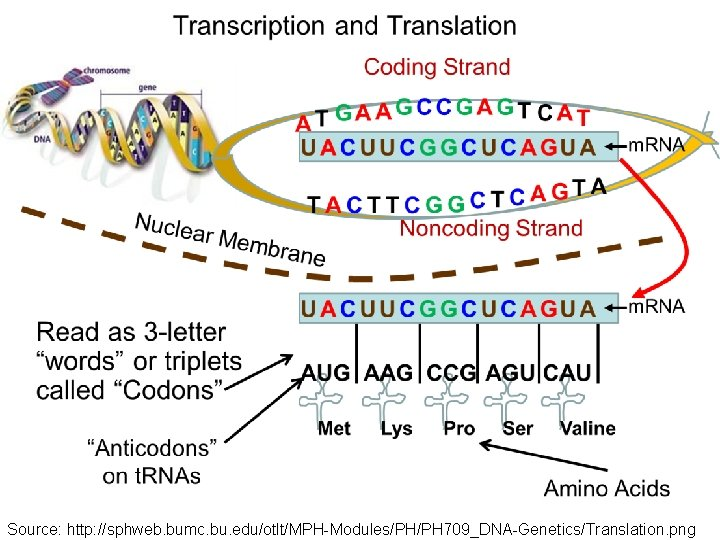Source: http: //sphweb. bumc. bu. edu/otlt/MPH-Modules/PH/PH 709_DNA-Genetics/Translation. pngExample of coded animal (now extinct) • Possible described only by protein order declaration • Disadvantage: evolution took three billion years, altho being massively parallel • Genotype -> fenotype translation example (extinct in cambrium; Kingdom: Animalia, Phylum: Euarthropoda (as insect or lobsters), Class: Dinocaridida) Source: www. as. wvu. edu/~kgarbutt/Evolution. Page/Studentsites/Burgesspages/images/opabinia. gif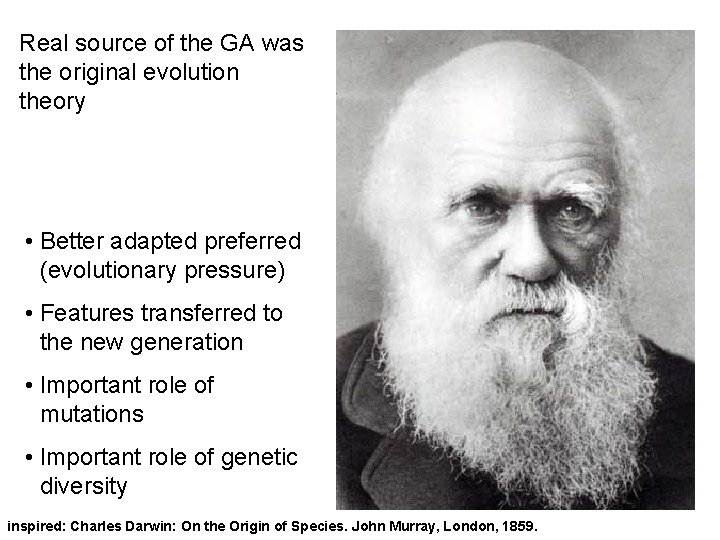Real source of the GA was the original evolution theory • Better adapted preferred (evolutionary pressure) • Features transferred to the new generation • Important role of mutations • Important role of genetic diversity inspired: Charles Darwin: On the Origin of Species. John Murray, London, 1859.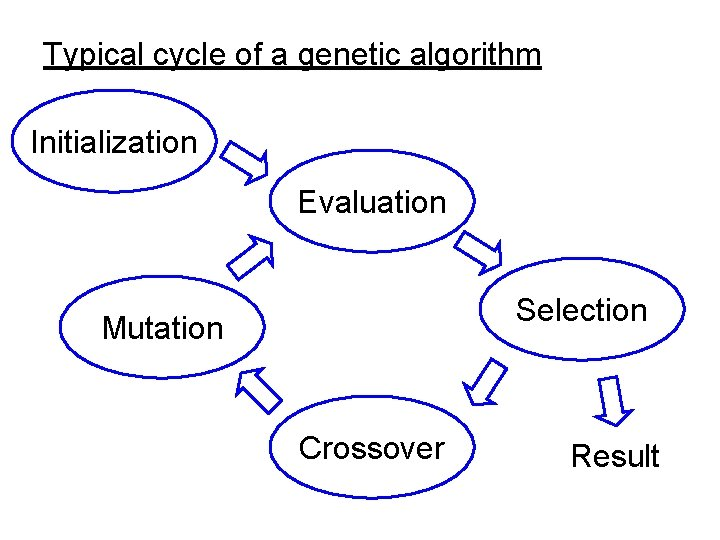Typical cycle of a genetic algorithm Initialization Evaluation Selection Mutation Crossover Result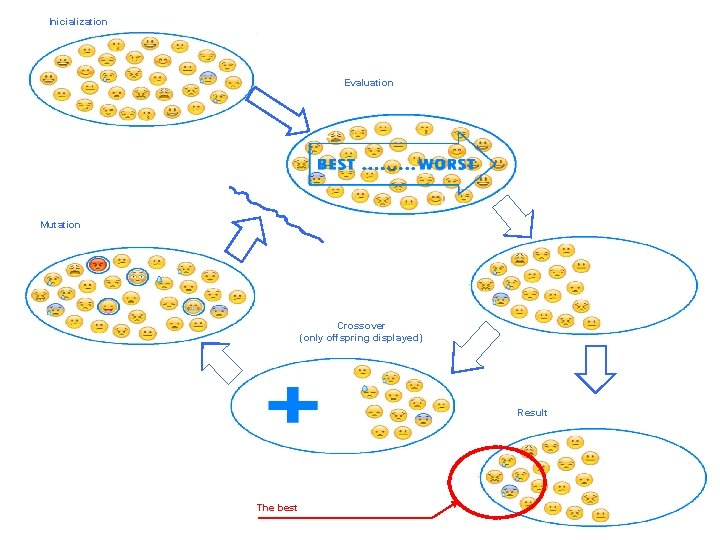Inicialization Evaluation Mutation Crossover (only offspring displayed) Result The best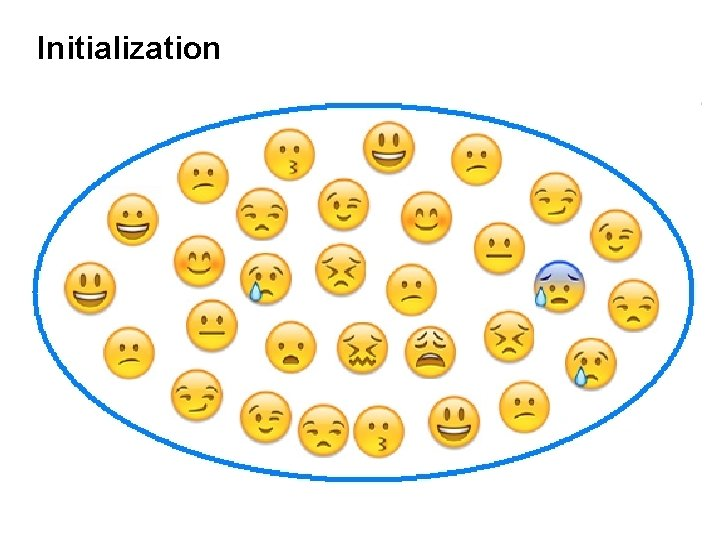Initialization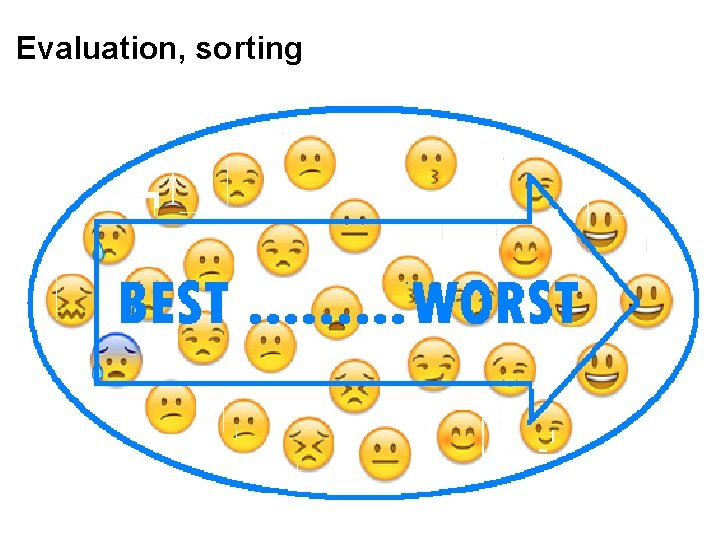Evaluation, sortingSelectionNew offspring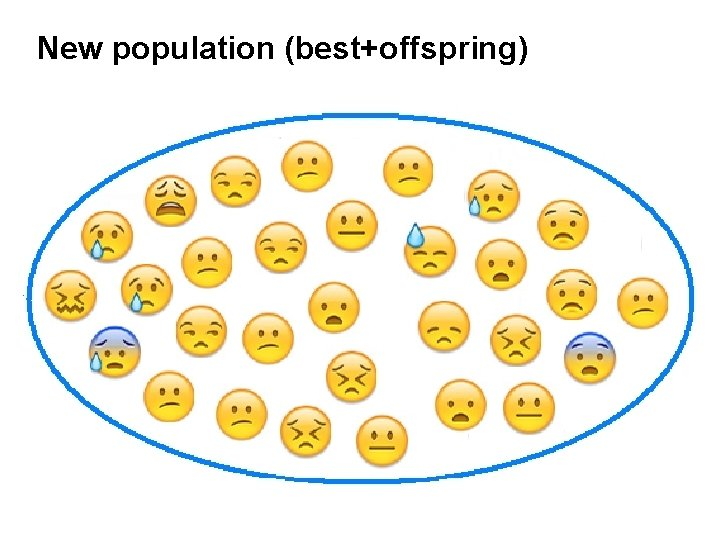New population (best+offspring)Mutation (marked individuals)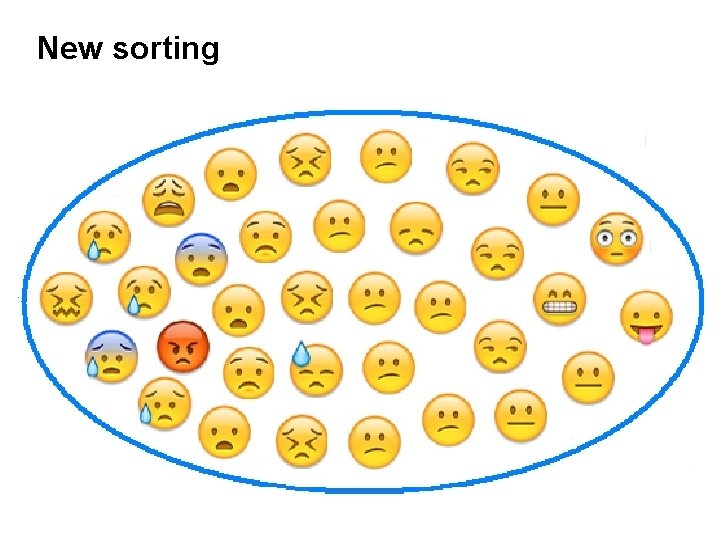New sorting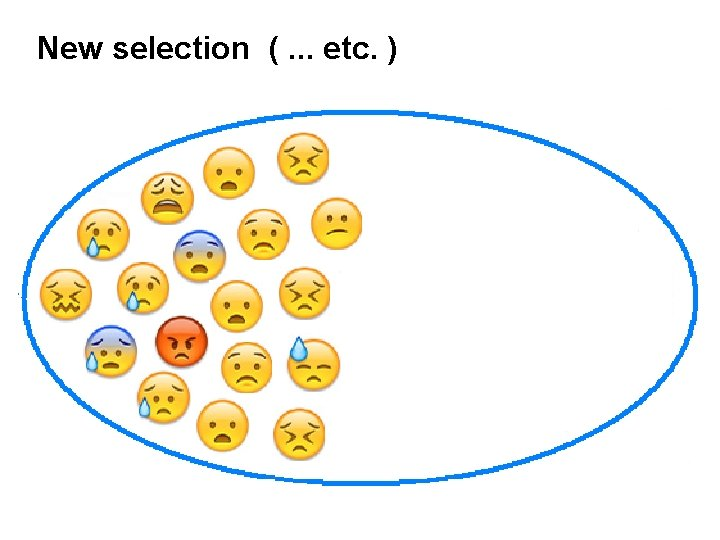New selection (. . . etc. )Note: only one of the mutated survive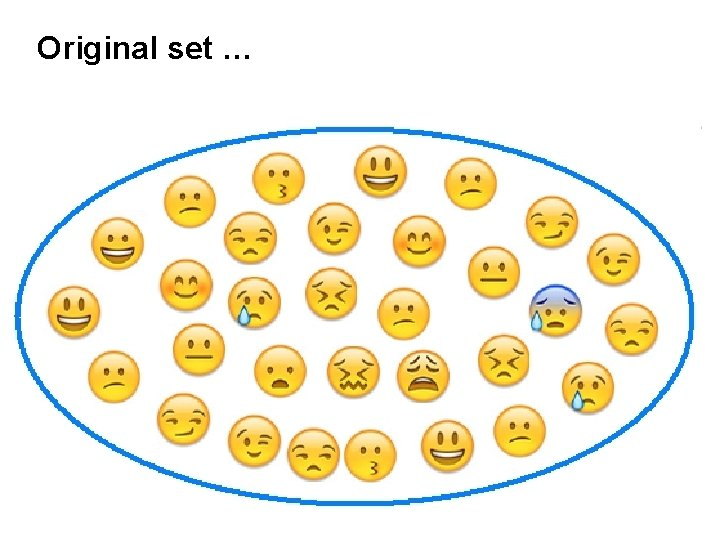Original set …… situation after one cycleCrossover example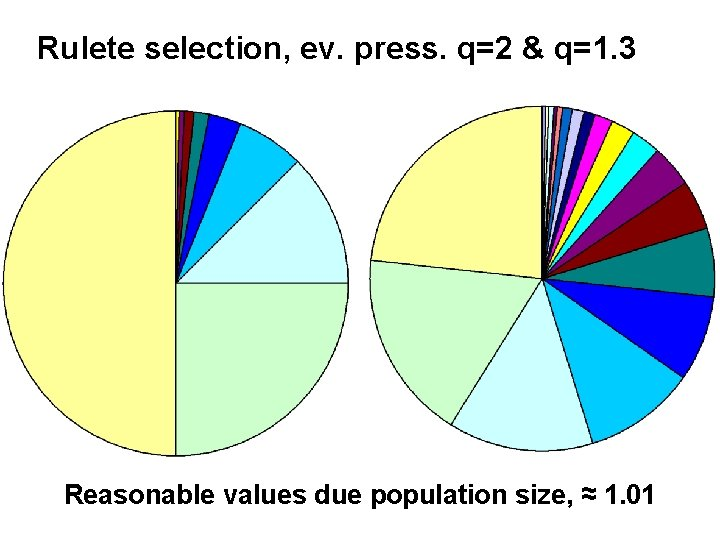Rulete selection, ev. press. q=2 & q=1. 3 Reasonable values due population size, ≈ 1. 01Real roulette wheal, all the compartments have the same size.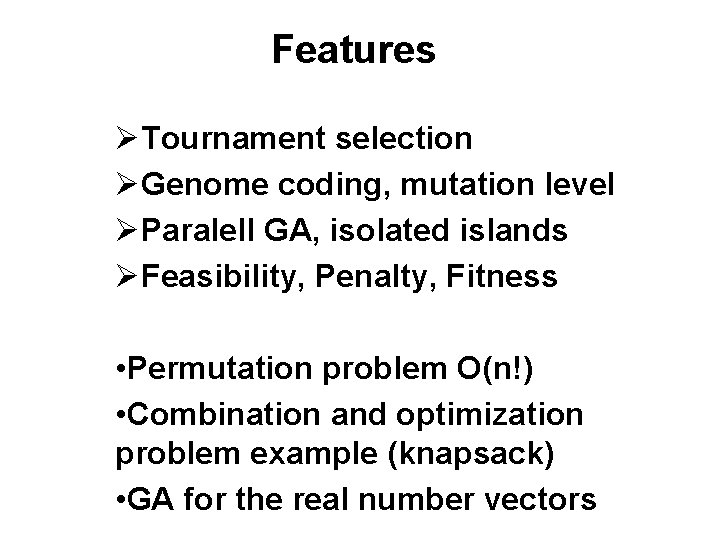Features ØTournament selection ØGenome coding, mutation level ØParalell GA, isolated islands ØFeasibility, Penalty, Fitness • Permutation problem O(n!) • Combination and optimization problem example (knapsack) • GA for the real number vectorsTraveling salesmanJeff Erickson: Algorithms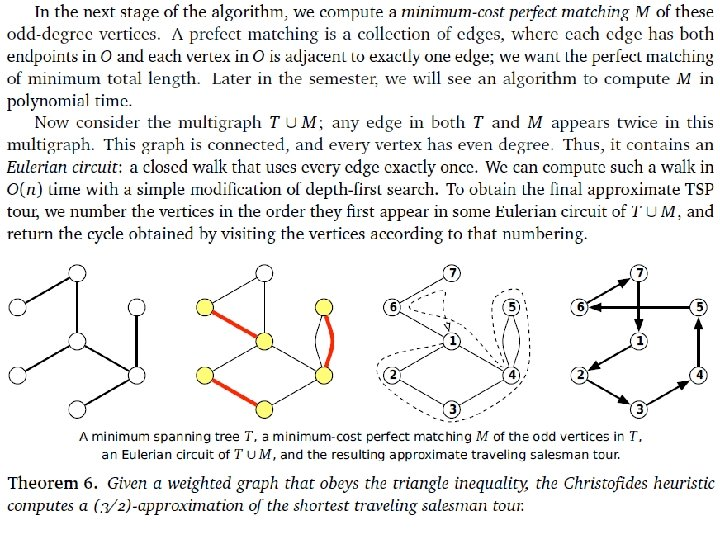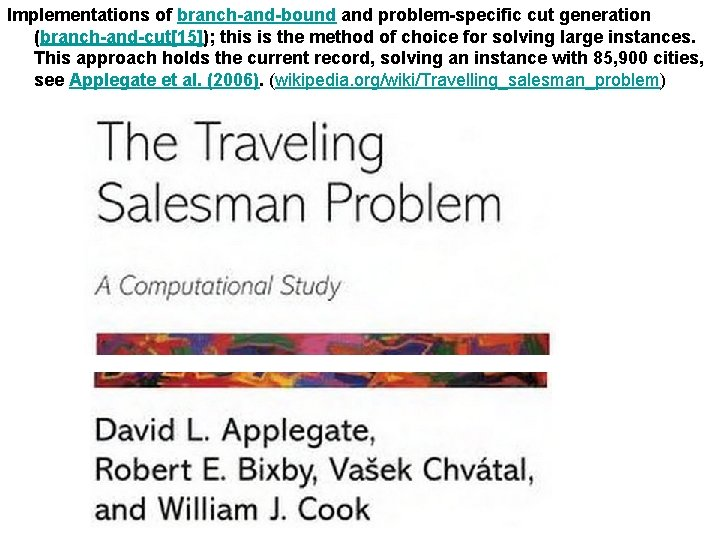Implementations of branch-and-bound and problem-specific cut generation (branch-and-cut); this is the method of choice for solving large instances. This approach holds the current record, solving an instance with 85, 900 cities, see Applegate et al. (2006). (wikipedia. org/wiki/Travelling_salesman_problem)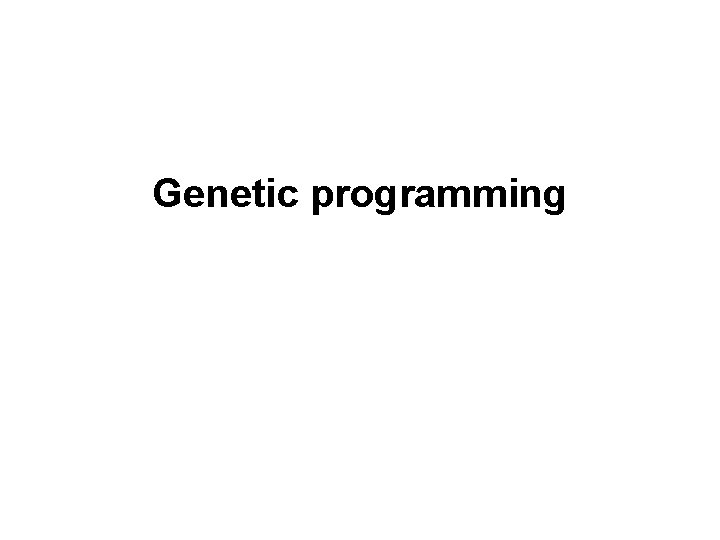Genetic programming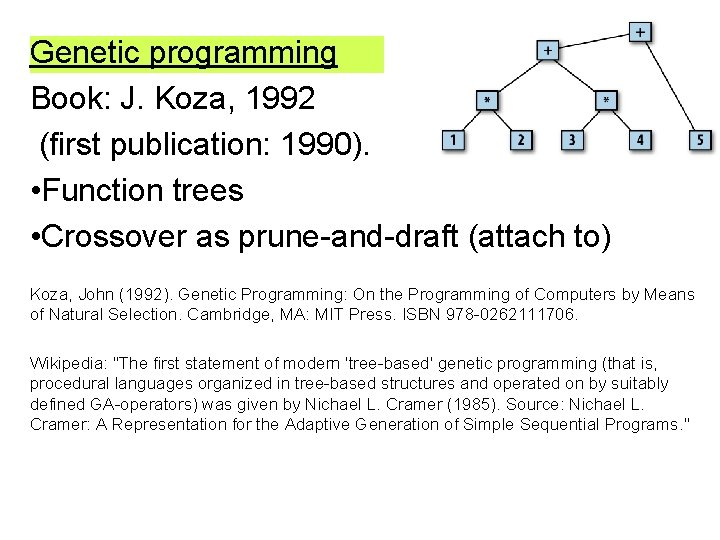Genetic programming Book: J. Koza, 1992 (first publication: 1990). • Function trees • Crossover as prune-and-draft (attach to) Koza, John (1992). Genetic Programming: On the Programming of Computers by Means of Natural Selection. Cambridge, MA: MIT Press. ISBN 978 -0262111706. Wikipedia: "The first statement of modern 'tree-based' genetic programming (that is, procedural languages organized in tree-based structures and operated on by suitably defined GA-operators) was given by Nichael L. Cramer (1985). Source: Nichael L. Cramer: A Representation for the Adaptive Generation of Simple Sequential Programs. "Use tree for the record of a function − a prefix: − a b infix: a−b postfix: a b − b (polish notation, poland math. Jan Łukasiewicz, 1920) (common, but parenthesis required) (HP 35; for 8087, the form of “ba-” is used)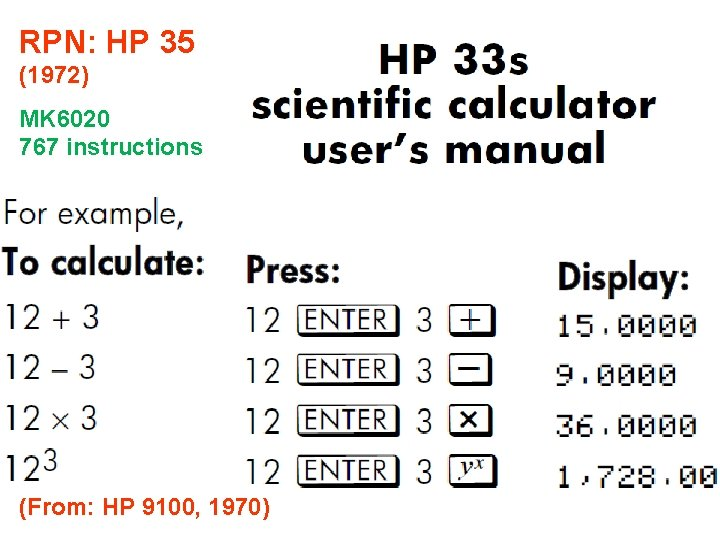RPN: HP 35 (1972) MK 6020 767 instructions (From: HP 9100, 1970)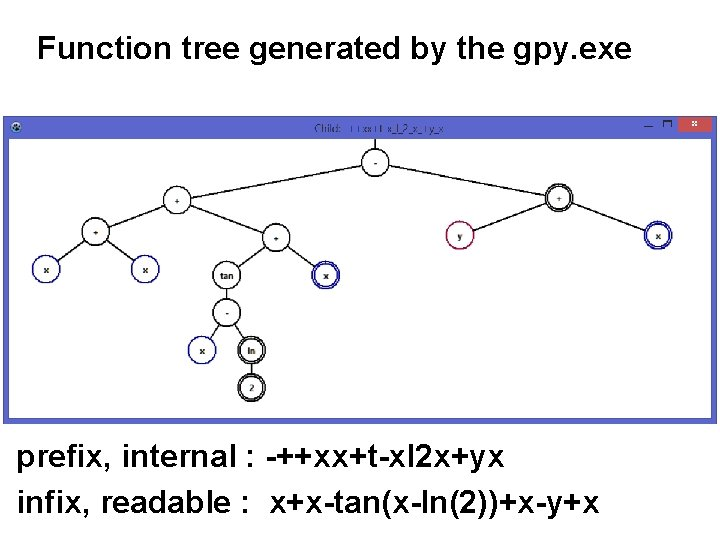Function tree generated by the gpy. exe prefix, internal : -++xx+t-xl 2 x+yx infix, readable : x+x-tan(x-ln(2))+x-y+x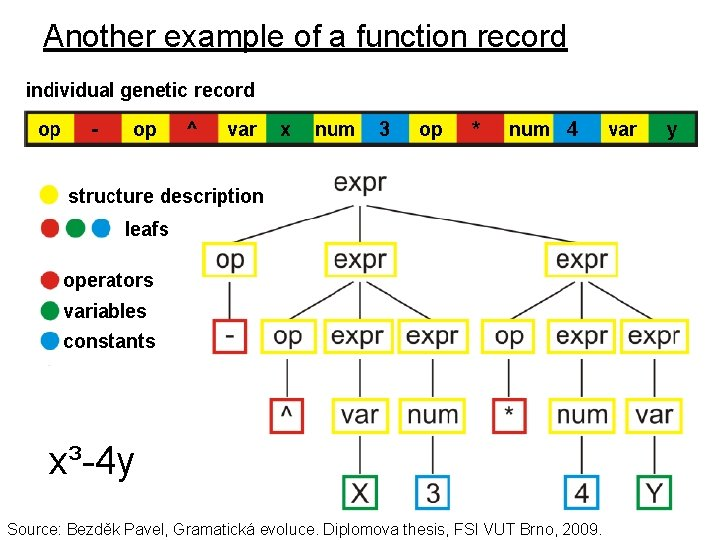Another example of a function record x³-4 y Source: Bezděk Pavel, Gramatická evoluce. Diplomova thesis, FSI VUT Brno, 2009.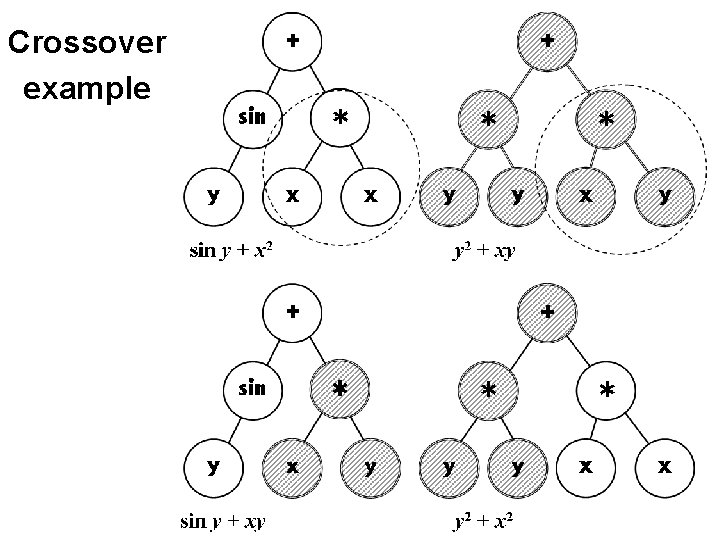Crossover example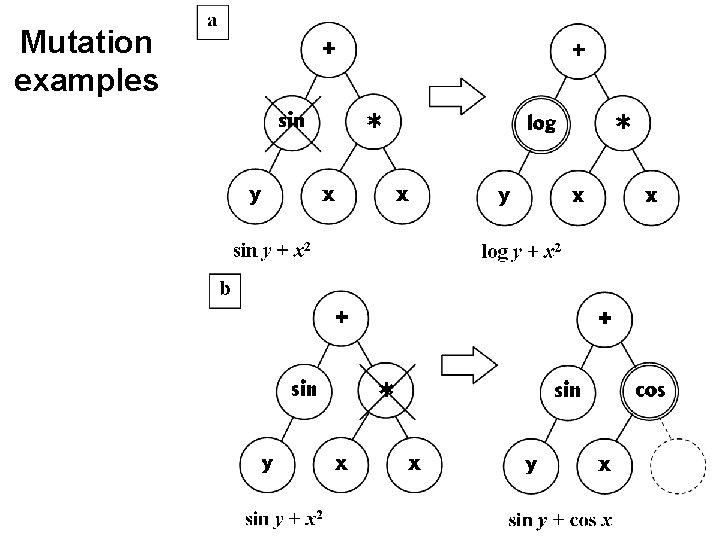Mutation examplesConstant representation – leaf in the graph, preset values: (Source: Banzhaf, W. , at al. : Genetic Programming, an Introduction. Page 138, figure 5. 11. Fugure 5. 12 on the next slide: )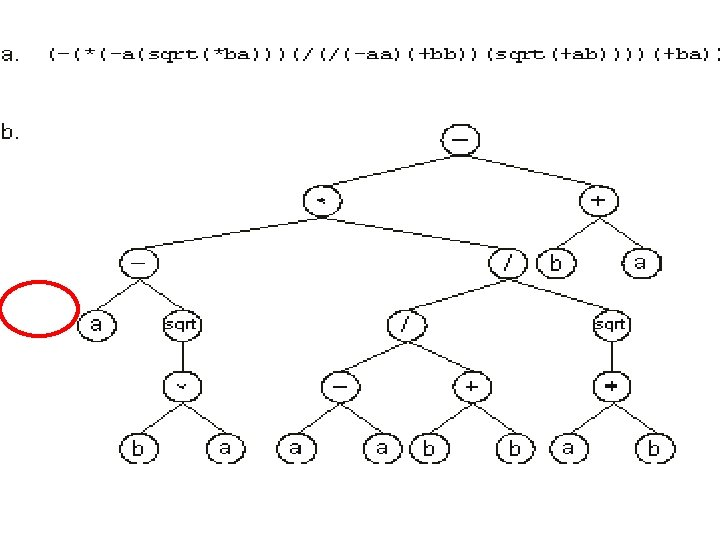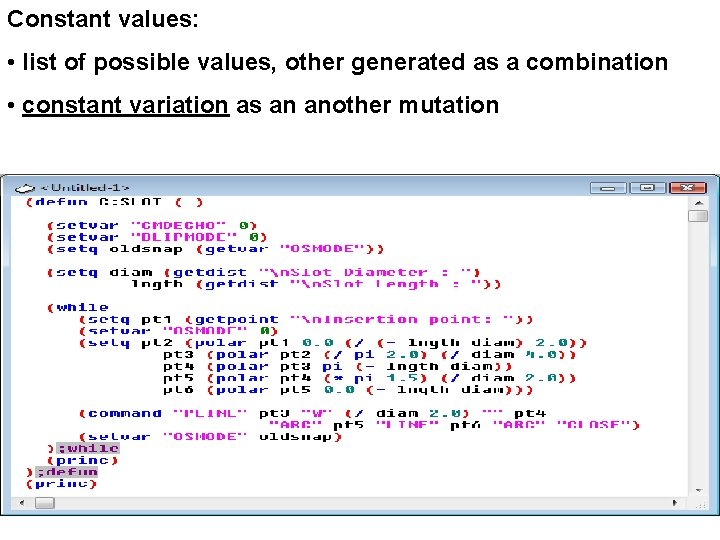Constant values: • list of possible values, other generated as a combination • constant variation as an another mutation(-(*(-a(sqrt(*ba)))(/(/(-aa)(+bb))(sqrt(+ab))))(+ba)) LISP (1958) *Grammatic evolution – the Backus-Naur form (Algol):Pascal, [Nicholas Wirth] - railroad diagram definitionPreselected list of constant values limits use of the GP for the symbolic regression. But how to evaluate them? [BT 4] Brandejsky T. : Genetic Programming Algorithm with constants pre-optimization of modified candidates of new population. In: Mendel 2004 • the best combination of the parameters (here: constants) are searched by the genetic algorithms with the real-coded genome (but described as GPA-ES, evolutionary strategies) For each of the newly generated individual (inside one step of the GP cycle), whole evolution process is run to get the correct parameter combination. Following picture is from: Brandejsky, T. : Evolutionary system to model structure and parameters regression. Neural Network World, 22 (2), pp. 181 -194, 2012.GA, ES, swarm intelligence: Advantages: • reliable while looks for parameter values • no extra conditions for search space (differentiable, etc. ) • required accuracy can be selected • there is no local minima problem Disadvantages: • often very slow • the search space of generated function is usually continuous • the overfitting problem, parameters can correct function, which is not the best for given data • it is easily possible to solve the local minima problem by the main GP cycle (constant variation method)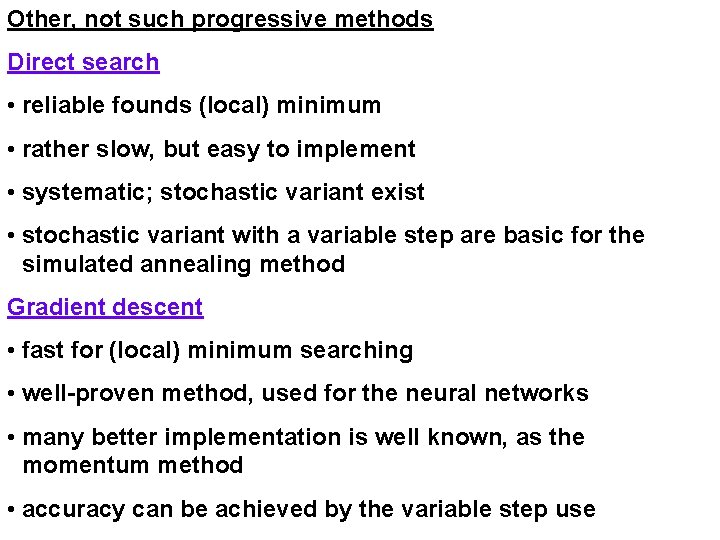Other, not such progressive methods Direct search • reliable founds (local) minimum • rather slow, but easy to implement • systematic; stochastic variant exist • stochastic variant with a variable step are basic for the simulated annealing method Gradient descent • fast for (local) minimum searching • well-proven method, used for the neural networks • many better implementation is well known, as the momentum method • accuracy can be achieved by the variable step use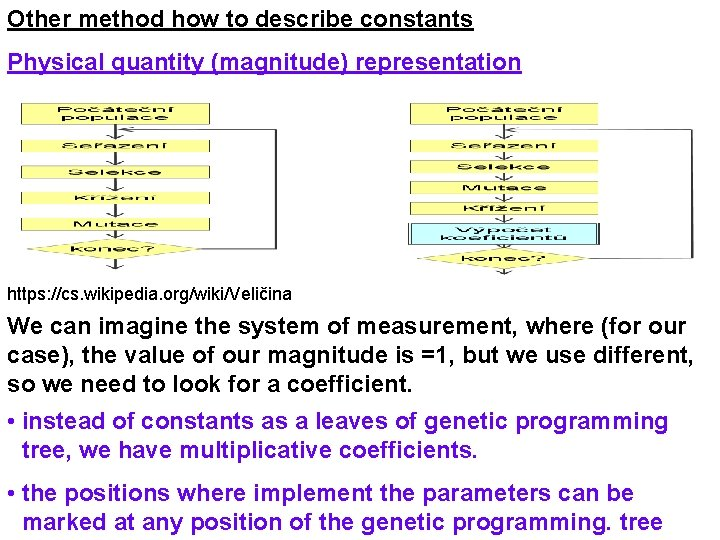Other method how to describe constants Physical quantity (magnitude) representation https: //cs. wikipedia. org/wiki/Veličina We can imagine the system of measurement, where (for our case), the value of our magnitude is =1, but we use different, so we need to look for a coefficient. • instead of constants as a leaves of genetic programming tree, we have multiplicative coefficients. • the positions where implement the parameters can be marked at any position of the genetic programming. tree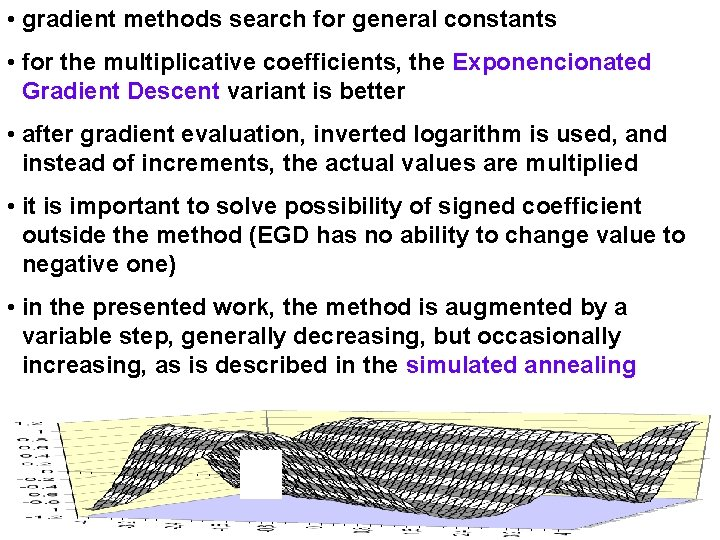• gradient methods search for general constants • for the multiplicative coefficients, the Exponencionated Gradient Descent variant is better • after gradient evaluation, inverted logarithm is used, and instead of increments, the actual values are multiplied • it is important to solve possibility of signed coefficient outside the method (EGD has no ability to change value to negative one) • in the presented work, the method is augmented by a variable step, generally decreasing, but occasionally increasing, as is described in the simulated annealing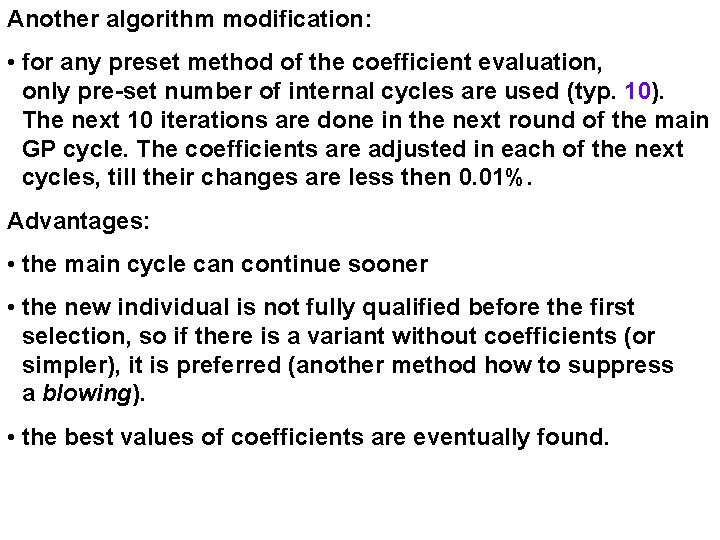Another algorithm modification: • for any preset method of the coefficient evaluation, only pre-set number of internal cycles are used (typ. 10). The next 10 iterations are done in the next round of the main GP cycle. The coefficients are adjusted in each of the next cycles, till their changes are less then 0. 01%. Advantages: • the main cycle can continue sooner • the new individual is not fully qualified before the first selection, so if there is a variant without coefficients (or simpler), it is preferred (another method how to suppress a blowing). • the best values of coefficients are eventually found.Zápis koeficientů do stromu • umístění koeficientů je zaznamenáno jako osmý bit označení uzlu (listu) stromu • maximálně deset koeficientů, při překročení počtu (například křížením) je program náhodně odstraňuje • koeficienty jsou zaznamenány v poli o deseti prvcích ke každému jedinci • pomalejší, ale univerzálnější řešení by byl záznam do spojového seznamu, který by nemusel být omezen maximálním počtem deset • většina vyhledaných úspěšných funkcí měla do pěti koeficientůTesting of proposed method • program was modified to choose a different method • the data are generated for a proposed function • to make condition more realistic, some noise is add. • an injection of possible solution is added to program • the record of the evolution is modified, so it is able to record the individual constant evaluation • the computing time is count in the unit of “how many function evaluation” is required. • for the graphs, five tests is done for each of the methods • the median of the resulting set is used as a representation of an individual method (time for getting 10% accuracy is compared)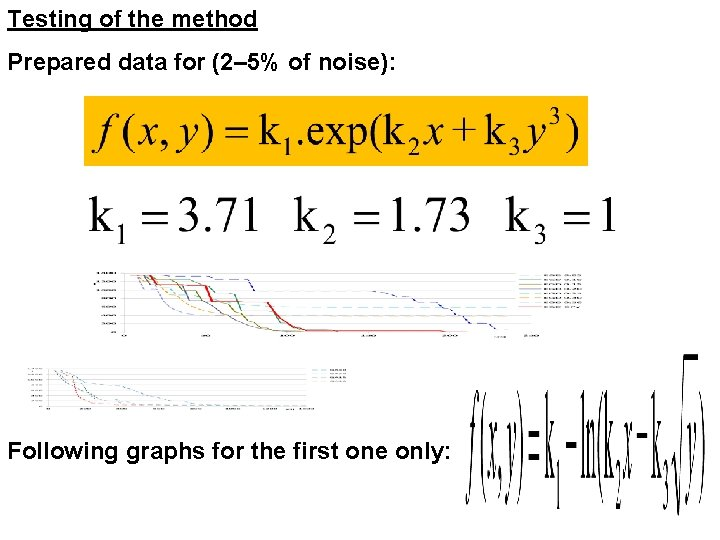Testing of the method Prepared data for (2– 5% of noise): Following graphs for the first one only: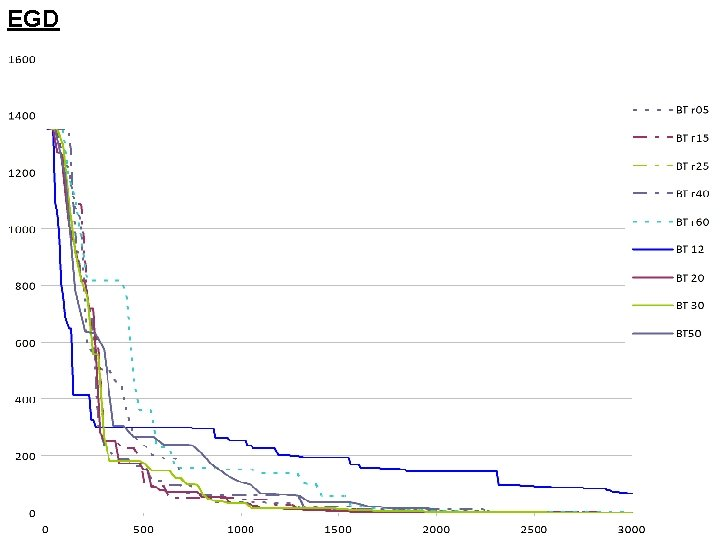EGD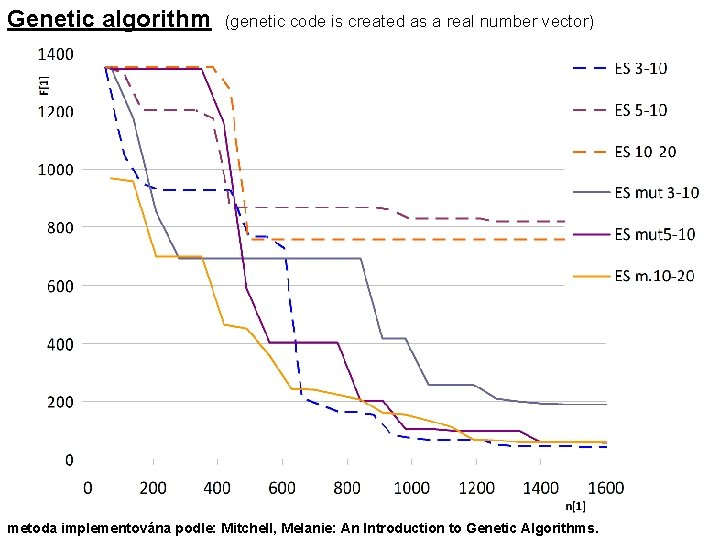Genetic algorithm (genetic code is created as a real number vector) metoda implementována podle: Mitchell, Melanie: An Introduction to Genetic Algorithms.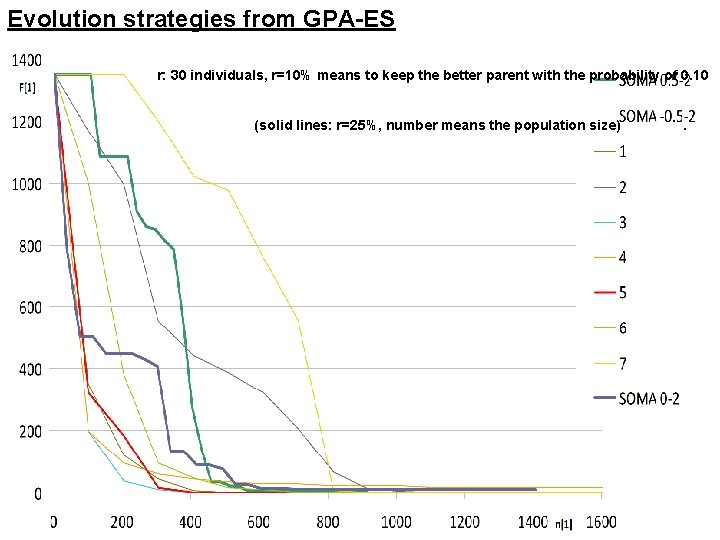Evolution strategies from GPA-ES r: 30 individuals, r=10% means to keep the better parent with the probability of 0. 10. . (solid lines: r=25%, number means the population size) .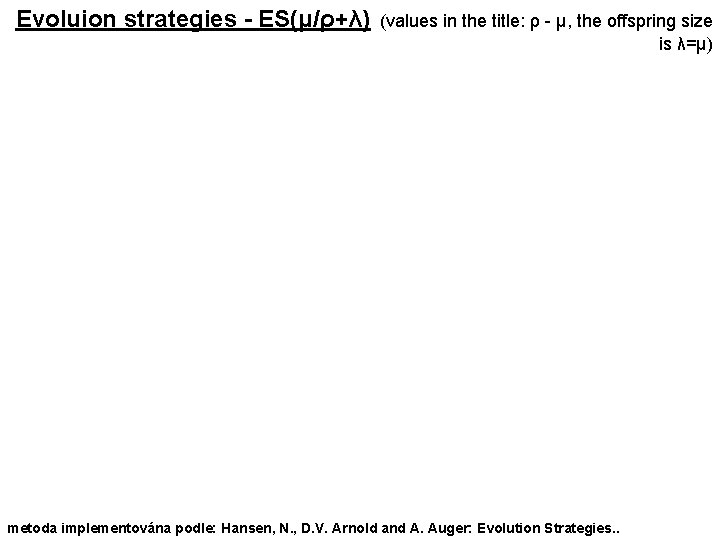Evoluion strategies - ES(μ/ρ+λ) (values in the title: ρ - μ, the offspring size is λ=μ) metoda implementována podle: Hansen, N. , D. V. Arnold and A. Auger: Evolution Strategies. .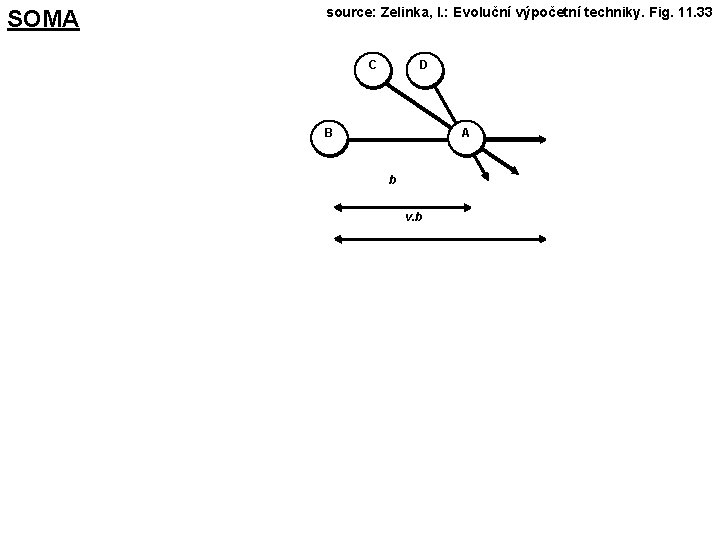SOMA source: Zelinka, I. : Evoluční výpočetní techniky. Fig. 11. 33 C D B A b v. bOverall comparison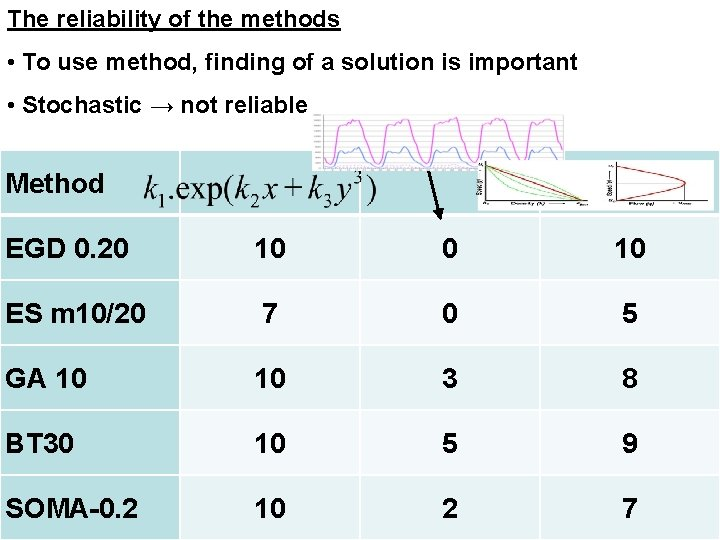The reliability of the methods • To use method, finding of a solution is important • Stochastic → not reliable Method EGD 0. 20 10 ES m 10/20 7 0 5 GA 10 10 3 8 BT 30 10 5 9 SOMA-0. 2 10 2 7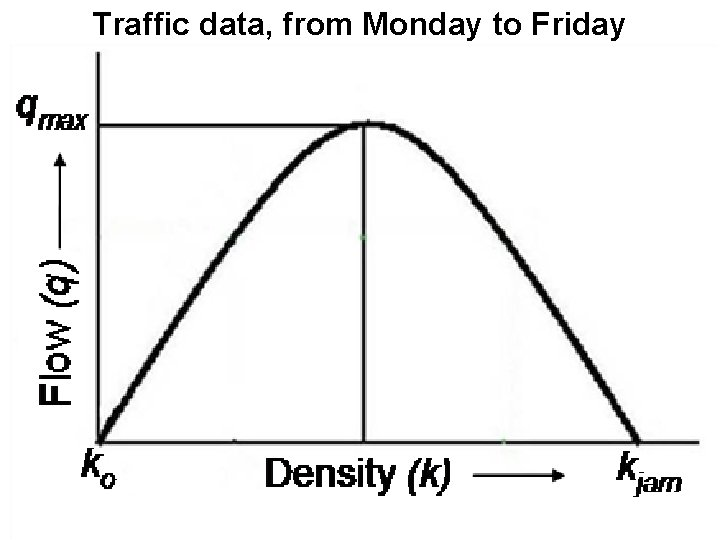Traffic data, from Monday to FridayTheoretical dependencies From: Venkatachalam, T. A. , Gnanavelu, D. : Concentration of Heterogeneous Road Traffic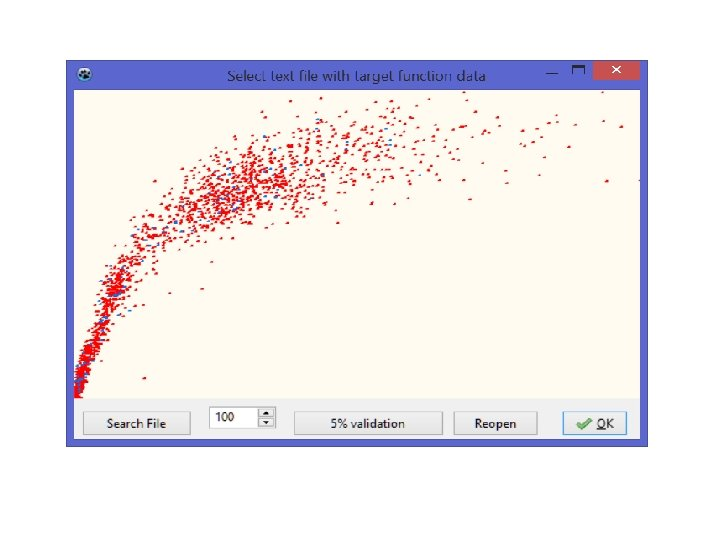3 D graph of the real dependency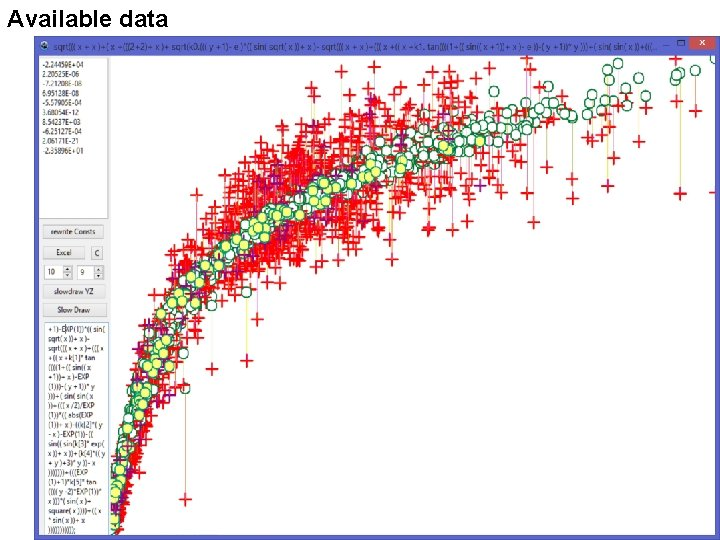Available dataAfter interrupt (press ESC, wait, …„Elapsed time: 21: 28: 02“)